# Electronics and Communication Engineering - Analog Electronics

36.

In figure, V0 =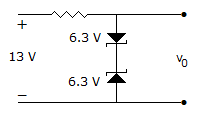A. zero B. 5.7 V C. 6.9 V D. 12.6 V

Explanation:

Output voltage is j 6.3 + 0.7 V.

37.

In the op-amp circuit of figure, V0 =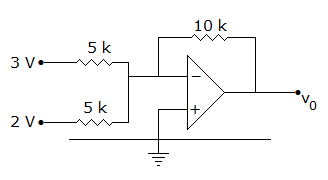A. 10 V B. -10 V C. 5 V D. -5 V

Explanation: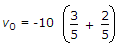38.

In figure the zener has a resistance of 5 ohms. As the load resistance is varied, the output voltage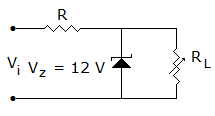A. will remain constant at 12 V B. will vary between 12.05 and 12.65 V C. will vary from 10 to 20 V D. will remain constant at 12.05 V

Explanation:

V0 = 12 + Iz x 5, Max Iz = 130 mA, minimum Iz = 10 mA.

39.

Percentage increase in the reverse saturation current of a diode if the temperature is increased from 25°C to 50°C

 A. 50% B. 500% C. 565.7% D. 575.6%

Explanation: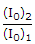= 2(T2 - T1)/10= 2(50-25)/10 x 100%565.7% .

40.

The transistor of following figure in Si diode with a base current of 40 μA and ICBO = 0, if VBB = 6V, RE = 2 kΩ and β = 90, IBQ = 20 μA then IEQ =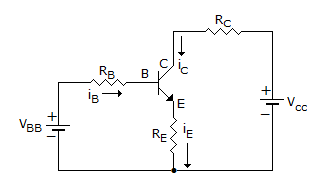A. 1800 μA B. 180 μA C. 2000 μA D. 3000 μA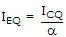, ICQ = β IBQ = 90 x 20 μA = 1800 μA.